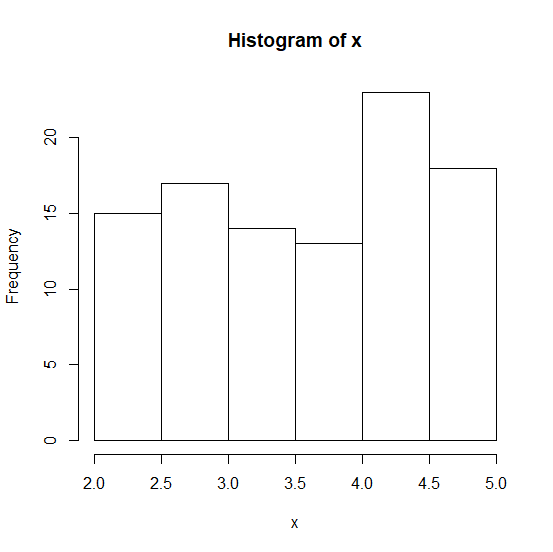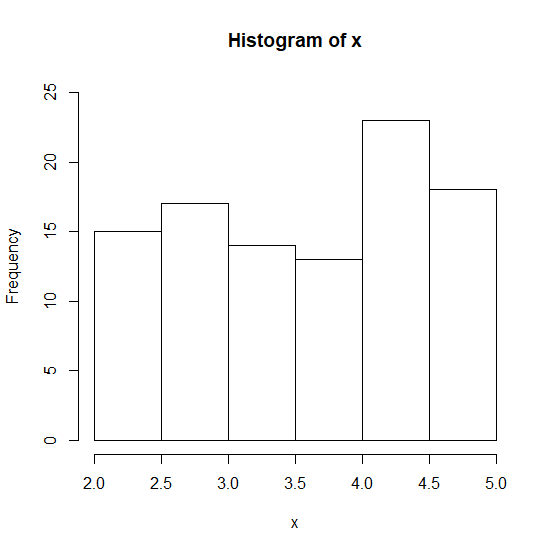# How to change the scale of Y-axis if the histogram bars are higher than the axis size in R?

When we create a histogram using hist function in R, often the Y-axis labels are smaller than the one or more bars of the histogram. Therefore, the histogram does not look appealing and it becomes a little difficult to match the Y-axis values with the bars size.

To solve this problem, we can use ylim argument of hist function in which the range can be supplied to plot on the Y-axis labels.

## Example

Consider the below data and its histogram −

Live Demo

set.seed(101)
x<-runif(100,2,5)
hist(x)

## OutputCreating the same histogram with higher Y-axis −

## Example

hist(x,ylim=c(0,25))


## Output